# Test: Operational Amplifiers

## 20 Questions MCQ Test GATE Electrical Engineering (EE) 2023 Mock Test Series | Test: Operational Amplifiers

Description
Attempt Test: Operational Amplifiers | 20 questions in 60 minutes | Mock test for Electronics and Communication Engineering (ECE) preparation | Free important questions MCQ to study GATE Electrical Engineering (EE) 2023 Mock Test Series for Electronics and Communication Engineering (ECE) Exam | Download free PDF with solutions
QUESTION: 1

### For the circuit shown in fig. the input resistance is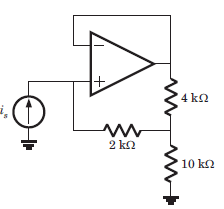Solution:

Since op-amp is ideal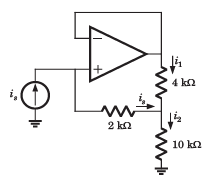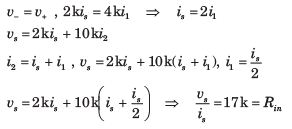QUESTION: 2

### In the circuit of fig. the op-amp slew rate is SR = 0.5 V/μs. If the amplitude of input signal is 0.02 V, then the maximum frequency that may be used is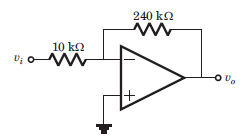Solution: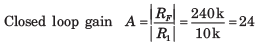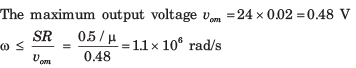QUESTION: 3

### In the circuit of fig. the input offset voltage and input offset current are Vio = 4 mV and Iio = 150 nA. The total output offset voltage is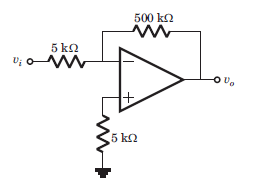Solution: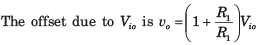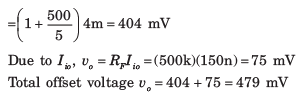QUESTION: 4

io = ?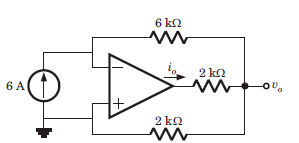Solution: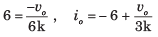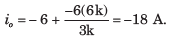QUESTION: 5

If ‘V’ is the voltage phasor and ‘I’ is the current phasor, then VI represents

Solution:

Apparent Power (S): It is defined as the product of r.m.s value of voltage (V) and current (1). It is denoted by S. S = V/I Volt Ampere

QUESTION: 6

Consider the circuit shown below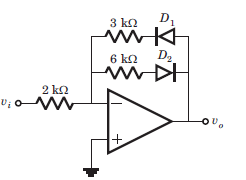Que: If vi = -2 V, then output vo is

Solution:

If vi < 0, then v0 > 0, D1 blocks and D2 conducts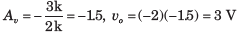QUESTION: 7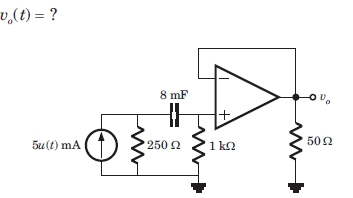Solution:

Voltage follower v0 = v = v+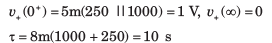QUESTION: 8

The circuit shown in fig. is at steady state before the switch opens at t = 0. The vc(t) for t > 0 is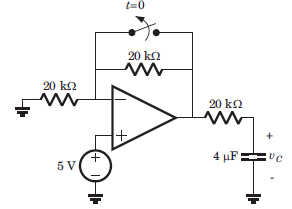Solution: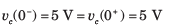For t > 0 the equivalent circuit is shown in fig.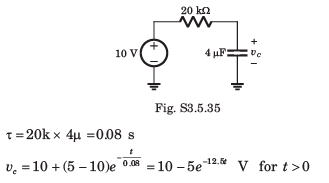QUESTION: 9

The LED in the circuit of fig. will be on if vis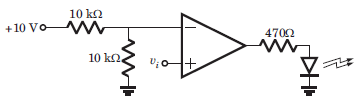Solution: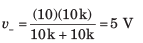When v+ > 5 V, output will be positive and LED will be on. Hence (C) is correct.

QUESTION: 10

In the circuit of fig. the CMRR of the op-amp is 60 dB. The magnitude of the vo is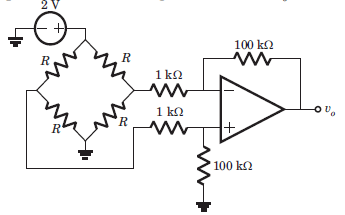Solution: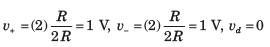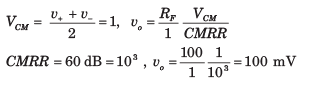QUESTION: 11

The analog multiplier X of fig. has the characteristics vp = v1v2 The output of this circuit is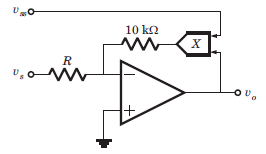Solution:

v+ = 0 = v-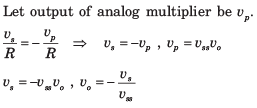QUESTION: 12

If the input to the ideal comparator shown in fig. is a sinusoidal signal of 8 V (peak to peak) without any DC component, then the output of the comparator has a duty cycle of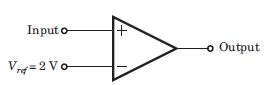Solution:

When vi > 2 V, output is positive. When vi < 2 V, output is negative.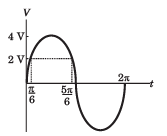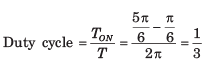QUESTION: 13

In the op-amp circuit given in fig. the load current iL is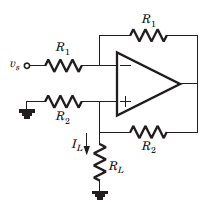Solution: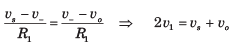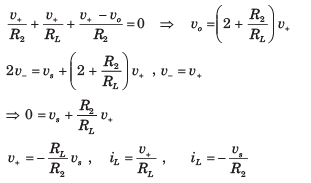QUESTION: 14

In the circuit of fig. output voltage is |vo| =1 V for a certain set of ω, R, an C. The |vo| will be 2 V if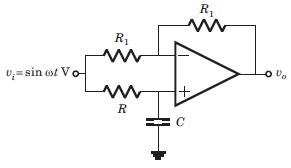Solution:

This is a all pass circuit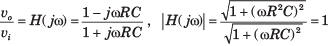Thus when ω and R is changed, the transfer function is unchanged

QUESTION: 15

In the circuit of fig. the 3 dB cutoff frequency is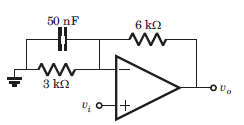Solution: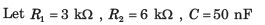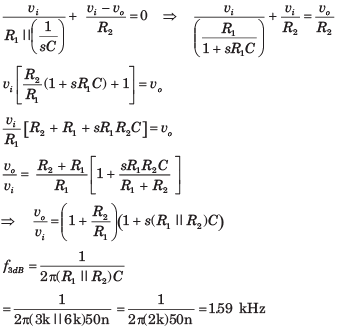QUESTION: 16

The phase shift oscillator of fig. operate at f = 80 kHz. The value of resistance RF is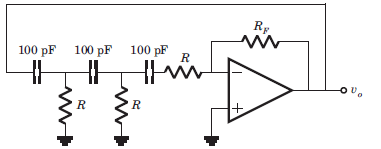Solution:

The oscillation frequency is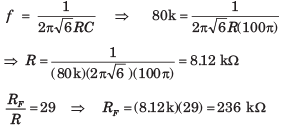QUESTION: 17

The value of C required for sinusoidal oscillation of frequency 1 kHz in the circuit of fig. is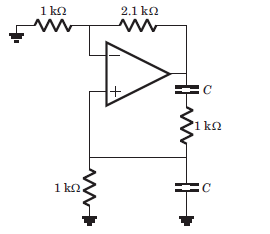Solution:

This is Wien-bridge oscillator. The ratio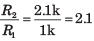is greater than 2. So there will be
oscillation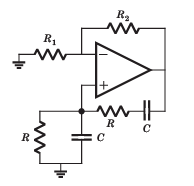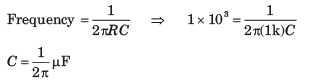QUESTION: 18

In the circuit shown in fig. the op-amp is ideal. If βF = 60, then the total current supplied by the 15 V source is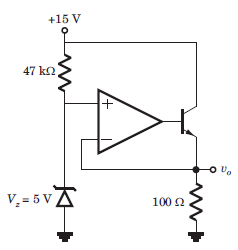Solution:

v+ = 5V = v = VE ,

The input current to the op-amp is zero.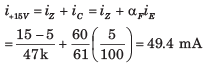QUESTION: 19

In the circuit in fig. both transistor Q1 and Q2 are identical. The output voltage at T = 300 K is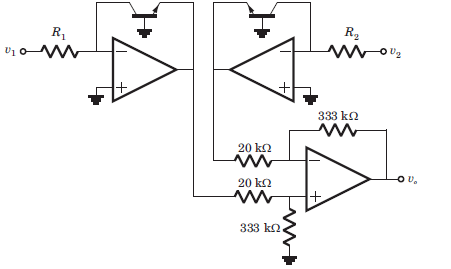Solution: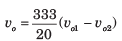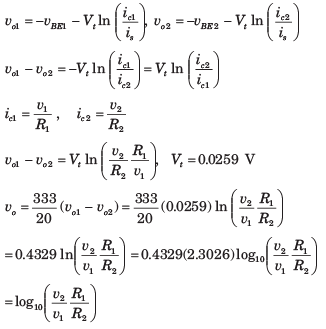QUESTION: 20

In the op-amp series regulator circuit of fig. Vz = 62. V, VBE = 0.7 V and β = 60. The output voltage vo is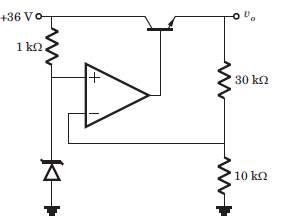Solution: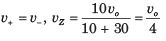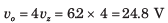Use Code STAYHOME200 and get INR 200 additional OFF Use Coupon Code